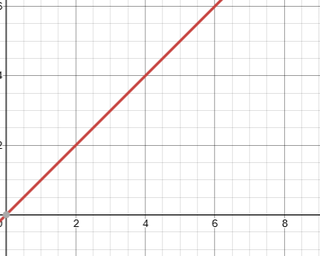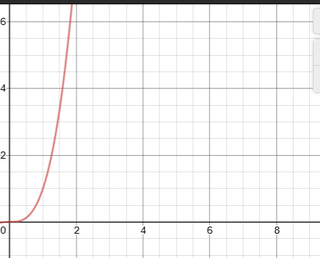Verified by Psychology Today

# It's Exponential

## Here is what it means for the coronavirus outbreak to be “exponential.”

From a statistical perspective, relationships among variables may take a broad array of forms. Sometimes, two variables may be unrelated to one another, such as (presumably) the scores on some college exam and attitudes about a particular pop star such as Justin Bieber.

Sometimes, two variables have a strong “linear” relationship. An accessible example of this might be the amount of time that a student studies for an exam as it relates to scores on the exam. Generally, speaking, more studying tends to be positively related to better exam scores. Further, we can conceptualize this pattern as a linear trend, as in Figure 1. Low scores on X (studying time) go with low scores on Y (exam score), while high scores on X (studying time) go with high scores on Y (exam score).Linear Function
Source: Glenn Geher

To create the function found in Figure 1, I simply went to a free online function creator (desmos.com) and put in the following equation: Y = X. This leads to a simple linear function.

Under some conditions, the relationship between two variables is not linear and is, rather, exponential in nature.

Sometimes, the relationship between two variables leads to an exponential function. This simply means that Y = some X variable raised to some exponent. So instead of Y = X (or, more formally, Y = 1*X, as in the prior example), we can imagine Y equaling some variable raised to some exponent. If you don’t recall this process from the math class that you may have taken years ago, I’ll explain. It’s easy.

Raising a variable to an exponent simply means that you multiply values of that variable by itself a certain number of times. If X = 3 and the function of interest is Y = X3, then Y = 33 (or three times itself three times). So 3*3*3, or 27. For the value of X = 4, we’d multiple 4 by itself 3 times: 4*4*4, for 64. You’ll see here the products go up much more quickly compared with the products in the linear equation used in Figure 1.Exponential Function
Source: Glenn Geher

Figure 2 is a graphical representation of this simple exponential function of Y = X3.

Notice how it differs from the function in Figure 1. The main difference is this: The curve goes up much more quickly and steeply for the exponential function compared with the curve for the linear function.

Now let’s think about what this means when scientists say that the spread of coronavirus follows an exponential function.

Pretty much, the function being exponential in nature simply means that the rate of contagion for corona is so high that the prevalence of cases in a population increases across time—with time being the X variable and prevalence of cases being the Y variable—in an exponential manner (much like in Figure 2).

A colloquial way of making this same point would be to say that this thing is spreading like wildfire (which tends to spread at an exponential rate).

Bottom Line

The world is a scary place right now. The coronavirus is changing everyday experiences across the globe. I was set to fly to SUNY Fredonia for an evaluation job in a few weeks; canceled; I was set to drive to New Jersey to tape a YouTube TV interview related to my work; canceled; I was set to give multiple presentations at a conference in Pittsburgh in early April; canceled … And that’s just me …

If there’s ever been an event in my lifetime that has been universally humbling and that has put all people in the same boat, it’s the coronavirus.

Hopefully, understanding what it means for this thing to have an exponential rate of contagion is helpful for people in realizing the magnitude of what all we are dealing with as a global community. To my mind, the World Health Organization (WHO) is the most expert, unbiased, and credible source of information on this situation. I say to check that website regularly, follow best practices and guidance found from the WHO, wash your hands regularly, limit contact with others, and stay safe.